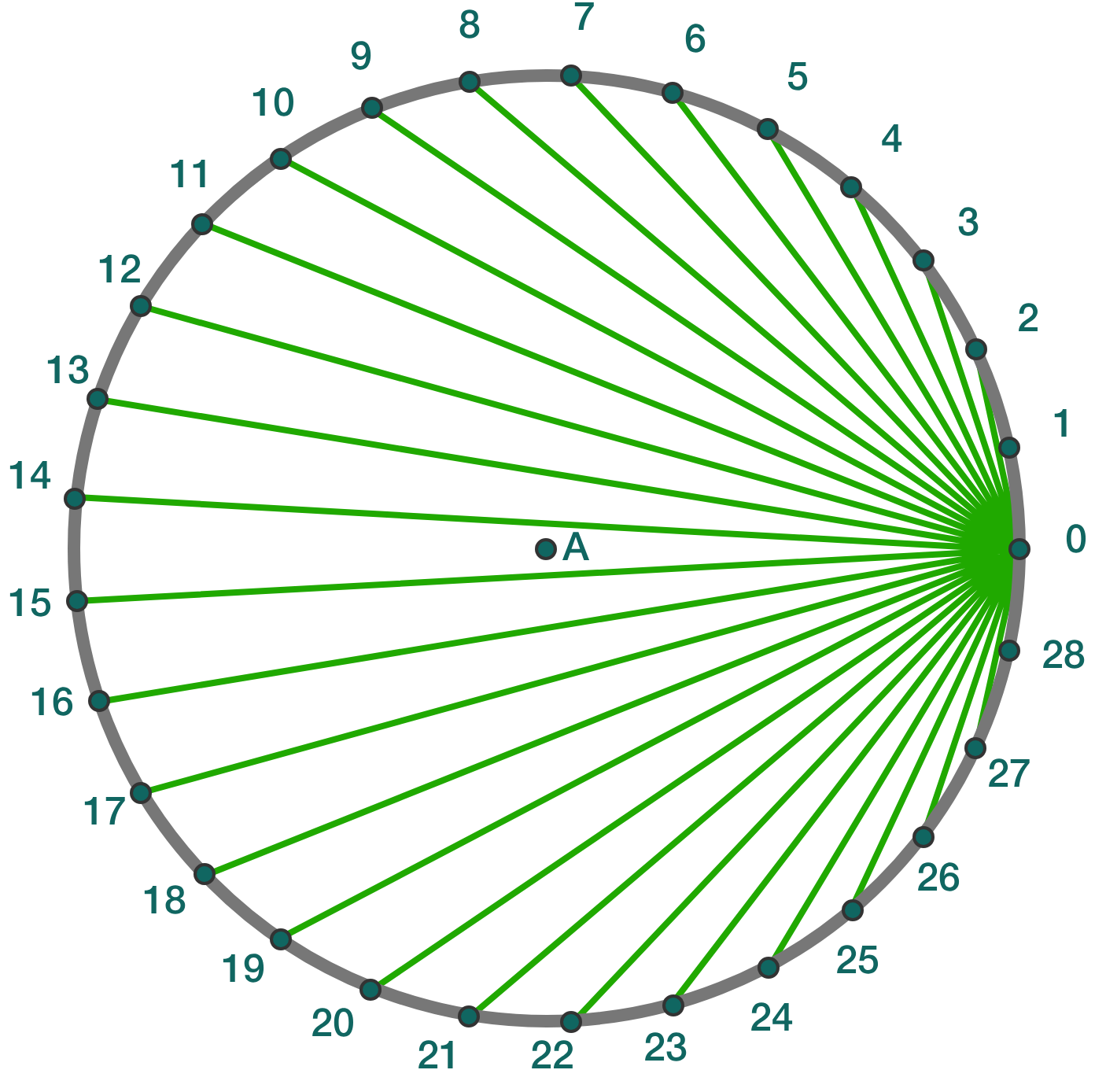# Problems of the Week

Contribute a problem

$2017$ is a prime number, so the sum of all its positive divisors is $1+2017= 2018.$

Is there another positive integer such that the sum of all its positive divisors is also $2018?$

$n=\sqrt{1+4\sqrt{1+5\sqrt{1+6\sqrt{1+\cdots }}}}$

What is the value of $n?$

The diagram shows 29 equidistant points (labeled 0,1,2,...,28) placed on a unit circle centered at point A.

Join the point $0$ to each of the other points, and let $x_k$ denote the length of the segment joining the points $0$ and $k.$

What is the value of $\displaystyle\left\lfloor 100\prod_{k=1}^{28} x_k \right\rfloor?$Notation: $\lfloor \cdot \rfloor$ denotes the floor function.

Given that $\displaystyle \pi = 4\sum_{k=1}^{\infty} \frac{\left(-1\right)^{k+1}}{2k-1},$ what is the value of

$\sum_{n=1}^{\infty} \left( \pi - 4\sum_{k=1}^{n} \frac{\left(-1\right)^{k+1}}{2k-1} \right)?$

Inspiration

$\sqrt { x\pm \sqrt { x\pm \sqrt { x\pm \sqrt { x\pm \cdots } } } }$

Within this infinitely nested radical, exactly 2018 of the $\pm$ symbols are assigned to be minus $(-)$ signs, with the rest of the $\pm$ symbols assigned to be plus $(+)$ signs (the minus signs don't need to be consecutive).

What's the minimum positive value of $x$ that guarantees the above expression has a real value?

Enter your answer in the form $\left\lfloor 1000x \right\rfloor.$

Note: Consider the worst-case scenario, among all possible places where the $2018$ minuses could be.

×